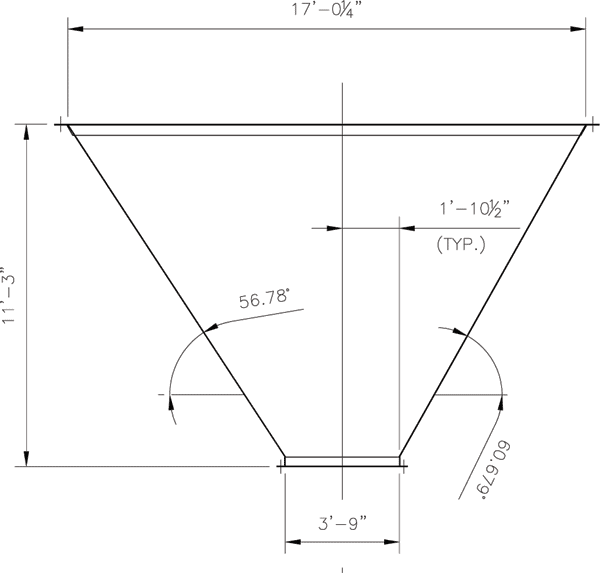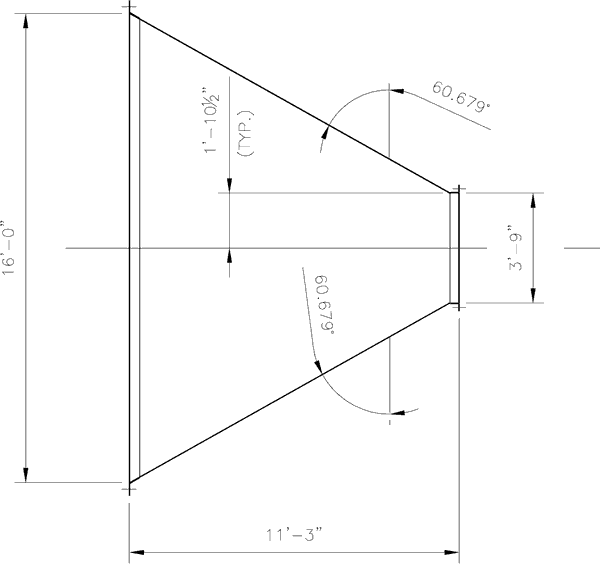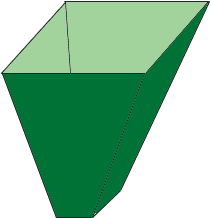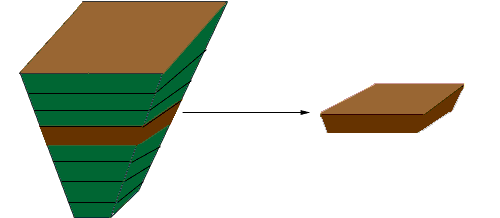Quandaries and Queries I would like to know the volume of this rectangular hopper….can you help….this would be a 10-12 level question. Hi Brian, Below are two of the diagrams you sent with the important measurements, and also a 3-D sketch of the hopper.I used calculus to find the volume. I am not going to go through the development but I want to give you an idea of how the volume is calculated. Suppose that the hopper is full of bread dough and you bake it to get a giant loaf of bread. Now slice it into horizontal slices.Each slice is rectangular on the top and bottom and the sides are slightly sloped. If the thickness of the slice is small you can approximate the volume of the slice by lengthwidththickness If you then add the volumes of the slices you get approximately the volume of the hopper. The calculus procedure then answers the question "What happens to this approximation of the volume of the hopper as the thickness of the slices approaches zero?" When I went through the calculations I got a volume of 1306.16 cubic feet. Penny Go to Math Central